Courses

# Doc: Events and Its Types- Probability Notes | EduRev

## JEE : Doc: Events and Its Types- Probability Notes | EduRev

The document Doc: Events and Its Types- Probability Notes | EduRev is a part of the JEE Course Mathematics For JEE.
All you need of JEE at this link: JEE

Events and its types

H. INDEPENDENT EVENTS

Two events A & B are said to be independent if occurrence or non occurrence of one does not effect the probability of the occurrence or non occurence of other.

(a) If the occurrence of one event affects the probability of the occurrence of the other event then the events are said to be dependent or Contingent. For two independent events A and B
P( A ∩ B) = P( A ) . P(B). Often this is taken as the definition of independent events.

(b) Three events A, B & C are independent if & only if all the following conditions hold ; P(A ∩ B) = P(A). P(B) ; P(B ∩ C) = P(B). P(C)
P(C ∩ A) = P(C). P(A) & P(A ∩ B ∩ C) = P(A). P(B). P(C)
i.e., they must be pairwise as well as mutually independent.
Similarly for n events A1, A2, A3, ........... An to be independent, the number of these conditions is equal to nC+ nC3 + ............. + nCn = 2n – n – 1.

Note : Independent events are not in general mutually exclusive & vice versa.
Mutually exclusiveness can be used when the events are taken from the same experiment & independence can be used when the events are taken from different experiments.

Ex.16 The probability that an anti aircraft gun can hit an enemy plane at the first, second and third shot are 0.6, 0.7 and 0.1 respectively. The probability that the gun hits the plane is

Sol. Let the events of hitting the enemy plane at the first, second and third shot are respectively A, B and C. Then as given P(A) = 0.6, P(B) = 0.7, P(C) = 0.1 Since events A, B, C are independent, so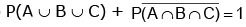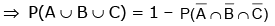Required probability =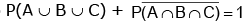= 1 – (1 – 0.6) (1 – 0.7) (1 – 0.1)) = 1 – (0.4)(0.3)(0.9) = 1 – 0.108 = 0.892

Ex.17 If two events A and B are such that P(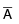) = 0.3 , P(B) = 0.4 and P(A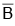) = 0.5 then P(B | (A ∪)) equals

Sol.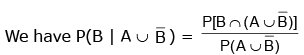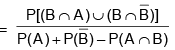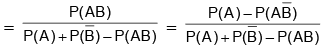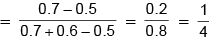I. PROBABILITY OF THREE EVENTS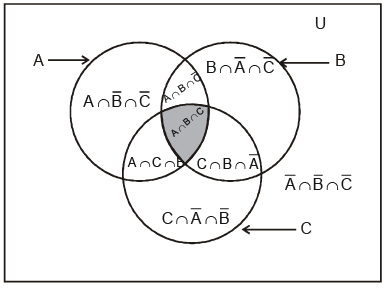For any three events A, B and C we have

(a) P(A or B or C) = P(A) + P(B) + P(C) – P(A ∩ B) – P(B ∩ C) – P(C ∩ A) + P(A ∩ B ∩ C)

(b) P (at least two of A, B, C occur) = P(B ∩ C) + P (C ∩ A) + P(A ∩ B) – 2P (A ∩ B ∩ C)

(c) P (exactly two of A, B, C occur) = P(B ∩ C) + P (C ∩ A) + P(A ∩ B) – 3P (A ∩ B ∩ C)

(d) P (exactly one of A, B, C occurs) = P(A) + P(B) + P(C) – 2P (B ∩ C) – 2P (C ∩ A) – 2P (A ∩ B) + 3P (A ∩ B ∩ C)

Note:  If there events A,B,C are pair wise mutually exclusive then they must be mutually exclusive i.e .P(A∩B) = P(B∩C) = P(C∩A) = 0 = P(A∩B∩C) = 0 .However the converse of this is not true

Offer running on EduRev: Apply code STAYHOME200 to get INR 200 off on our premium plan EduRev Infinity!

## Mathematics For JEE

123 videos|273 docs|299 tests

,

,

,

,

,

,

,

,

,

,

,

,

,

,

,

,

,

,

,

,

,

;